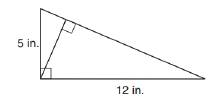Chapter 8.1, Problem 47EElementary Geometry For College St...

7th Edition
Alexander + 2 others
ISBN: 9781337614085

Solutions

Chapter
SectionElementary Geometry For College St...

7th Edition
Alexander + 2 others
ISBN: 9781337614085
Textbook Problem

In Exercises 47 to 50, use the fact that the area of the polygon is unique.In the right triangle, find the length of the altitude drawn to the hypotenuse.To determine

To find:

The length of the altitude drawn to the hypotenuse.

Explanation

Converting theoretical statement into mathematical statement, identifying the geometric figure and the calculation is done by suitable geometric formulae.

Calculation:

Given,

The lengths of the sides are,

AB=a=5 in

BC=b=12 in

Area of the right ΔABC(A)=12ab unit2.

=12(5)(12)

=(5)(6)

A=30 in2

Find AC:

For right triangle ABC

Here AB=5 in and BC=12 in

By Pythagorean theorem,

(AB)2+(BC)2=(AC)2

(5)2+(12)2=(AC)2

Still sussing out bartleby?

Check out a sample textbook solution.

See a sample solution

The Solution to Your Study Problems

Bartleby provides explanations to thousands of textbook problems written by our experts, many with advanced degrees!

Get Started

Find the distance between (2, 4) and (6, 8).

Applied Calculus for the Managerial, Life, and Social Sciences: A Brief Approach

Find all possible real solutions of each equation in Exercises 3144. y3+64=0

Finite Mathematics and Applied Calculus (MindTap Course List)

The graph of f(x) = 3x5 − 5x3 is:

Study Guide for Stewart's Single Variable Calculus: Early Transcendentals, 8th

True or False: is a convergent series.

Study Guide for Stewart's Multivariable Calculus, 8th